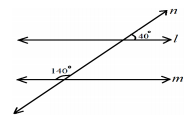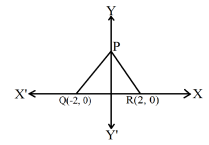# CBSE Class 9 Mathematics Sample Paper Set 58

Read and download PDF of CBSE Class 9 Mathematics Sample Paper Set 58 designed as per the latest curriculum and examination pattern for Class 9 issued by CBSE, NCERT and KVS. The latest Class 9 Mathematics Sample Papers have been provided with solutions so that the students can solve these practice papers and then compare their answers. This will help them to identify mistakes and improvement areas in Mathematics Class 9 which they need to study more to get better marks in Class 9 exams. After solving these guess papers also refer to solved Class 9 Mathematics Question Papers available on our website to build strong understanding of the subject

## Sample Paper for Class 9 Mathematics Pdf

Students can refer to the below Class 9 Mathematics Sample Paper designed to help students understand the pattern of questions that will be asked in Class 9 exams. Please download CBSE Class 9 Mathematics Sample Paper Set 58

### Mathematics Class 9 Sample Paper

SECTION – A

(Question numbers 1 to 4 carry 1 mark each)

Q.1. Write the rationalising factor or conjugate factor of √7 + √2.

Q.2. Write an example of a binomial of degree 35.

Q.3. Can a triangle have two obtuse angles? Give reason for your answer.

Q.4. How many axes and quadrants are there in a Cartesian plane?

SECTION – B

(Question numbers 5 to 10 carry 2 marks each)

Q.5. Write two rational numbers between 2/3 and 5/3 .

Q.6. Find the remainder when x4 + x3 – 2x2 + x + 1 is divided by x – 1.

Q.7. If B lies between A and C, AC = 15 cm and BC = 9 cm. What is ?

Q.8. In the given figure, can we say l || m, state with reasons?Q.9. In the given figure, ΔPQR is an equilateral triangle with coordinates of Q and R as (-2, 0) and (2, 0) respectively. Find the coordinates of the vertex P.Q.10. What will be the semi perimeter of an equilateral triangle of side ‘a’? Show your work.

## More Study Material

### Sample Paper Mathematics Class 9

We hope you liked the above provided CBSE Class 9 Mathematics Sample Paper Set 58. To get an understanding of the type of questions which were asked in exams, it is important for Class 9 students to refer to CBSE Class 9 Mathematics Sample Paper Set 58 that we have provided above. Students can download the Sample Paper for Class 9 Mathematics which will be coming in the exams so that you can practise them and solve all types of questions that can be asked in exams. If you do CBSE Class 9 Mathematics Sample Paper Set 58 for Class 9 Mathematics you will easily understand the way examination Sample Paper are set by teachers.

### Class 9 Mathematics Sample Paper

The latest Sample Paper CBSE Class 9 Mathematics Sample Paper Set 58 covers all the topics which have been suggested by CBSE in their Class 9 Mathematics syllabus for the current year. By doing CBSE Class 9 Mathematics Sample Paper Set 58 you will understand the regular questions and MCQ questions for Class 9 Mathematics which are always asked and learn them so that you can get full marks in those questions.

We have provided link above of CBSE Class 9 Mathematics Sample Paper Set 58 in Pdf format so that you can just click the links and easily download the papers with answers. On studiestoday, we have also provided a download and print option for all CBSE Class 9 Mathematics latest Sample Papers for Class 9 Mathematics to make it more easy for students. You can download CBSE Class 9 Mathematics Sample Paper and Class 9 Mathematics Question Papers in PDF absolutely free of cost.

#### Mathematics Sample Paper Class 9 with Solutions

We have provided CBSE Class 9 Mathematics Sample Paper Set 58 with solutions. You will be able to understand the type of answers which you should write in Class 9 Mathematics exams to score good marks. You should attempt all the last year question paper for Class 9 and Class 9 Mathematics MCQ Test in examination conditions at home and then compare their answers with the solutions provided by our teachers. This way you will be able to identify your weak areas and also understand how the teachers of Class 9 Mathematics set their questions in the exams.

#### CBSE Class 9 Mathematics Sample Paper with solutions

We have provided the current year Class 9 Mathematics Sample Paper. Students who want to practice Sample Paper from all previous years can easily get them on studiestoday. We are the leading website in India from which you can download all the latest Class 9 Mathematics study material for free as per the CBSE syllabus for Class 9 Mathematics for the current academic year.

Where can I download CBSE Class 9 Mathematics Sample Paper Set 58 in Pdf

You can download CBSE Class 9 Mathematics Sample Paper Set 58 from StudiesToday.com

Can I download the latest Sample Paper of Class 9 Mathematics in Pdf

Is the Class 9 Mathematics Mock Paper available for the latest session

Yes, the CBSE Class 9 Mathematics Sample Paper Set 58 issued for Class 9 Mathematics have been made available here for latest academic session

How can I download and print CBSE Class 9 Mathematics Sample Paper Set 58

You can easily access the link above and download CBSE Class 9 Mathematics Sample Paper Set 58 and save on your computer of mobile

Is there any charge for CBSE Class 9 Mathematics Sample Paper Set 58

There is no charge for CBSE Class 9 Mathematics Sample Paper Set 58 you can download everything free

How can I improve my scores by solving CBSE Class 9 Mathematics Sample Paper Set 58

Regular practice of sample question paper given on studiestoday for CBSE Class 9 Mathematics Sample Paper Set 58 can help you to score better marks in exams

Are there any websites that offer free CBSE Class 9 Mathematics Sample Paper Set 58

Yes, studiestoday.com provides all latest CBSE Class 9 Mathematics Sample Papers with answers based on the latest format issued for current academic session

Can model paper CBSE Class 9 Mathematics Sample Paper Set 58 be accessed on mobile devices

Yes, studiestoday provides CBSE Class 9 Mathematics Sample Paper Set 58 in Pdf and can be accessed on smartphones and tablets.

Are mock CBSE Class 9 Mathematics Sample Paper Set 58 available in multiple languages

Yes, mock CBSE Class 9 Mathematics Sample Paper Set 58 are available in multiple languages, including English, Hindi